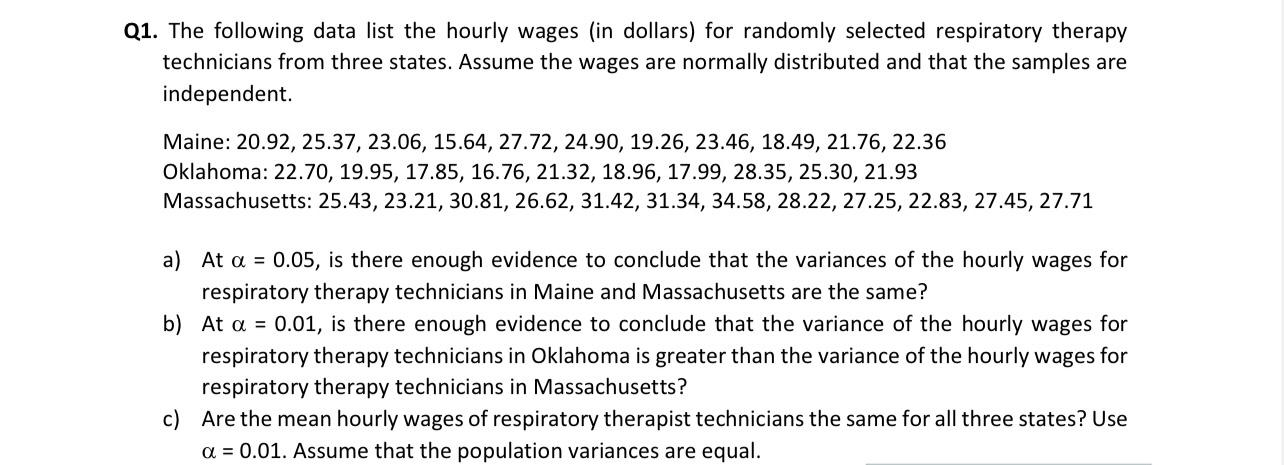Home / Expert Answers / Statistics and Probability / q1-the-following-data-list-the-hourly-wages-in-dollars-for-randomly-selected-respiratory-therap-pa256

# (Solved): Q1. The following data list the hourly wages (in dollars) for randomly selected respiratory therap ...Q1. The following data list the hourly wages (in dollars) for randomly selected respiratory therapy technicians from three states. Assume the wages are normally distributed and that the samples are independent. Maine: $$20.92,25.37,23.06,15.64,27.72,24.90,19.26,23.46,18.49,21.76,22.36$$ Oklahoma: 22.70, 19.95, 17.85, 16.76, 21.32, 18.96, 17.99, 28.35, 25.30, $$21.93$$ Massachusetts: $$25.43,23.21,30.81,26.62,31.42,31.34,34.58,28.22,27.25,22.83,27.45,27.71$$ a) At $$\alpha=0.05$$, is there enough evidence to conclude that the variances of the hourly wages for respiratory therapy technicians in Maine and Massachusetts are the same? b) At $$\alpha=0.01$$, is there enough evidence to conclude that the variance of the hourly wages for respiratory therapy technicians in Oklahoma is greater than the variance of the hourly wages for respiratory therapy technicians in Massachusetts? c) Are the mean hourly wages of respiratory therapist technicians the same for all three states? Use $$\alpha=0.01$$. Assume that the population variances are equal.

We have an Answer from Expert